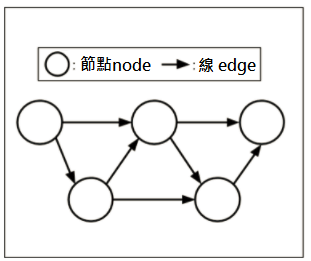## 技術部落格

// 【GCP 教學】如何在 GCP 上使用 TensorFlow?
a# 開源機器學習系統 TensorFlow 應用實例

## 什麼是 TensorFlow?

2017/12現在版本已經更新至1.4了，在教學文件上可以看到，能夠安裝的作業系統為 Linux 及 Mac OS X，開發語言為 C++ 及 Python 可供選擇。在本篇，作業系統會使用 Ubuntu 14.04 ，開發語言以Python 構築環境。

## 安裝 TensorFlow 的順序

 OS Ubuntu14.04LTS Machine type n1-standard-2 (2 vCPUs, 7.5 GB memory) CPU platform Intel Ivy Bridge Boot disk and local disks [Size (GB)] 10 Boot disk and local disks [Type] SSD persistent disk Boot disk and local disks [Mode] Boot, read/write

### 準備實際的環境

#### • 確認 Python 的版本

TensorFlow僅支援 Python 2.7及 3.3 版本集以上，下面指令可以執行確認：

``````\$ python -V
``````

#### • 安裝 pip 和 virtualenv

``````\$ sudo apt-get install python-pip python-dev python-virtualenv
``````

*1) pip 為管理 Python 軟體的 package
*2) virtualenv 為建立執行 Python 的虛擬環境的 package

#### • 實作 virtualenv 虛擬環境

``````\$ virtualenv --system-site-packages ~/tensorflow
``````

#### • 啟動讀取虛擬環境

``````\$ source ~/tensorflow/bin/activate
``````

``````(tensorflow)yasue@tensorflow-report:~\$
``````

### 於虛擬環境下安裝 TensorFlow

TensorFlow 的安裝非常簡單。TensorFlow 需要安裝讓 pip 可以動作的 *3) wheel 檔案。這個檔案於官方網站有提供。Wheel 檔案已經以 Python package 的形式包裝起來。最新的 TensforFlow Wheel 檔案的指定方法請參閱：https://www.tensorflow.org/install/install_linux

``````(tensorflow)yasue@tensorflow-report:~\$ pip install --ignore-install
``````

### 在 TensorFlow 上說 “Hello, TensorFlow!”

``(tensorflow)yasue@tensorflow-report:~\$ python``
``````import tensorflow as tf
# (1)
hello = tf.constant('Hello, TensorFlow!')
# (2)
sess = tf.Session()
print(sess.run(hello))

Hello, TensorFlow!
``````

(1) 使用 tf.constant() 就能製作 Tensor 類別的物件。這對大家來說或許比較少見，Tensor 類別是處理如多維度矩陣的類別。由於前述產生的「Hello, TensorFlow!」是文字，和矩陣比較無關。試試看輸入 Tensor 類別的變數 hello 看看：

``````print hello

Tensor("Const:0", shape=(), dtype=string)
``````

•
「Const」是「常數」
•
「shape」值為空白，維度為 0 之意
•
「dtype」為「string」字串，值的資料型態為文字的關係

(2) 接著建立「tf.Session()」，利用「print(sess.run(hello))」將 Tensor 類別的變數 hello 代入 Session 內開始計算。用「print()」將文字列印出來。

``````# (3)
a = tf.constant(10)
b = tf.constant(32)
# (4)
print(sess.run(a + b))

42
``````

### 順帶一提 – 什麼是 Dataflow Graph?TensorFlow 的計算可說分為以下 2 個階段處理：
1. 建構 Dataflow graph
2. 將 Dataflow graph 代入 Session 中，開始實際計算

## iKala Cloud

This site is registered on wpml.org as a development site.